Question

Suppose you are involved in designing a hypersonic missile. For the first test flight, the missile...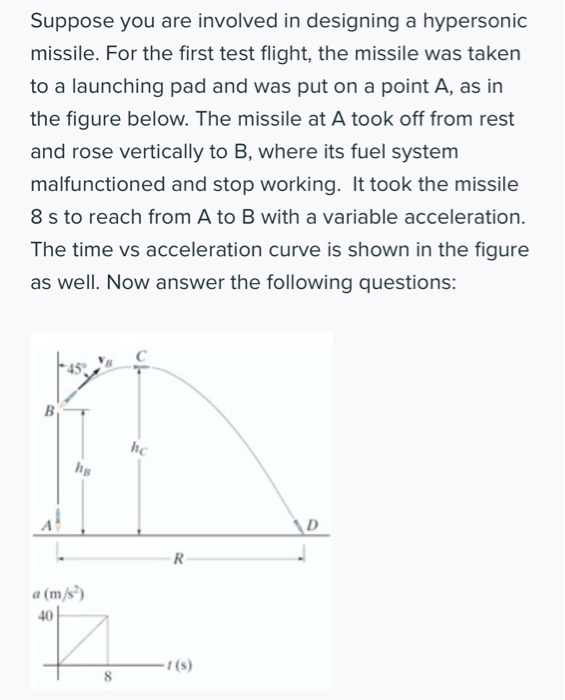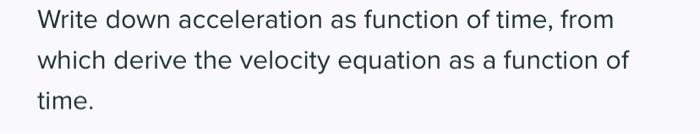Suppose you are involved in designing a hypersonic missile. For the first test flight, the missile was taken to a launching pad and was put on a point A, as in the figure below. The missile at A took off from rest and rose vertically to B, where its fuel system malfunctioned and stop working. It took the missile 8 s to reach from A to B with a variable acceleration. The time vs acceleration curve is shown in the figure as well. Now answer the following questions: a (m/s)
Write down acceleration as function of time, from which derive the velocity equation as a function of time.
Determine the missile's speed VB and height /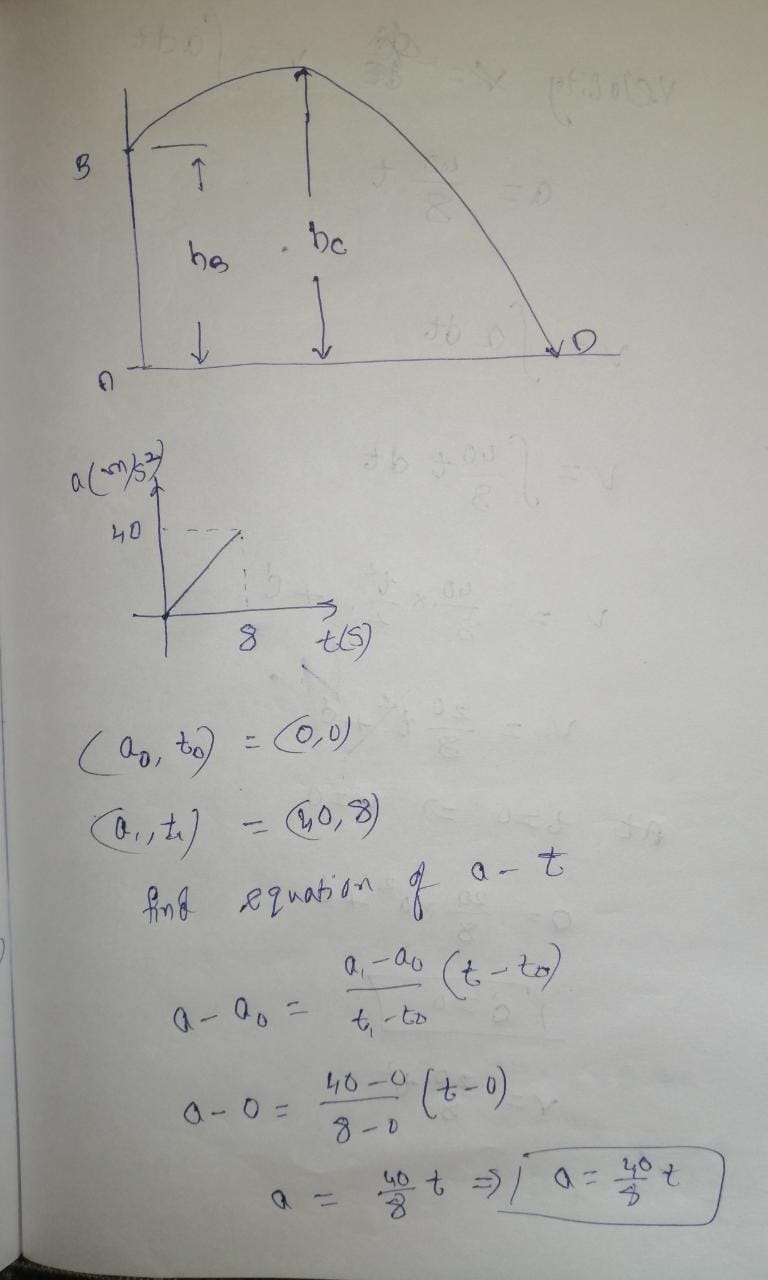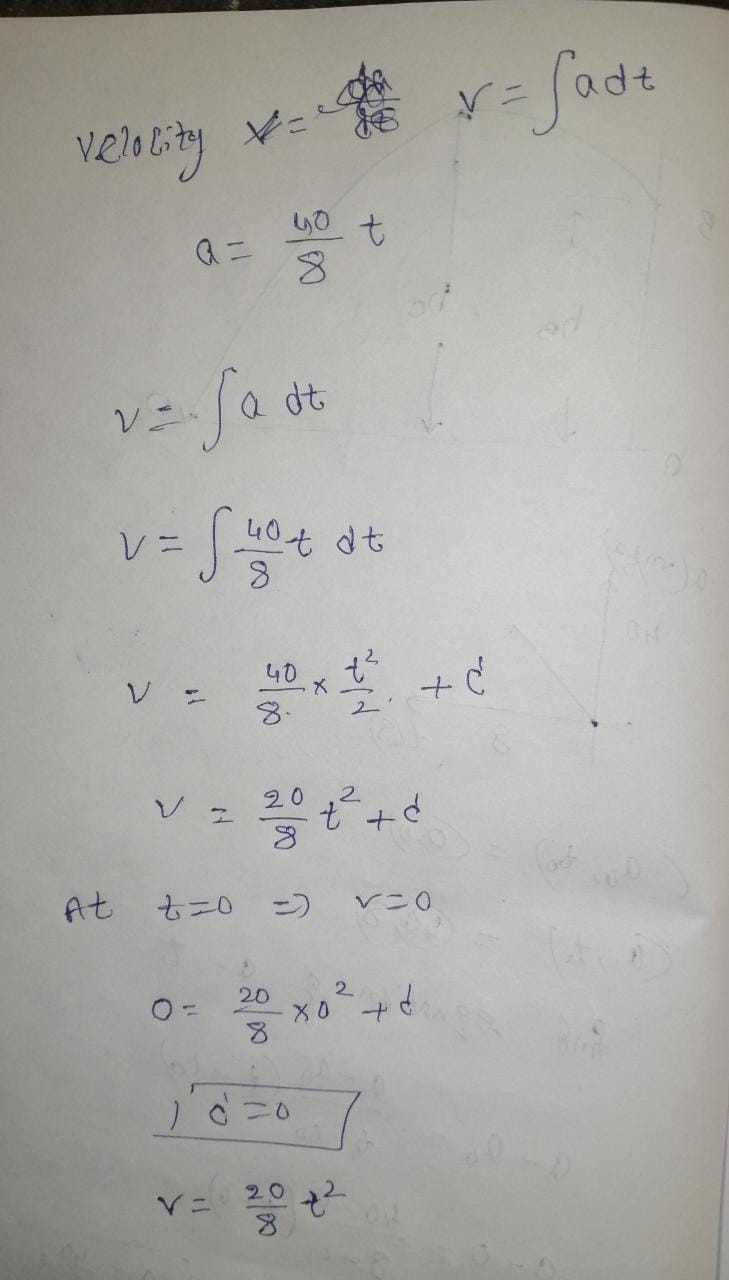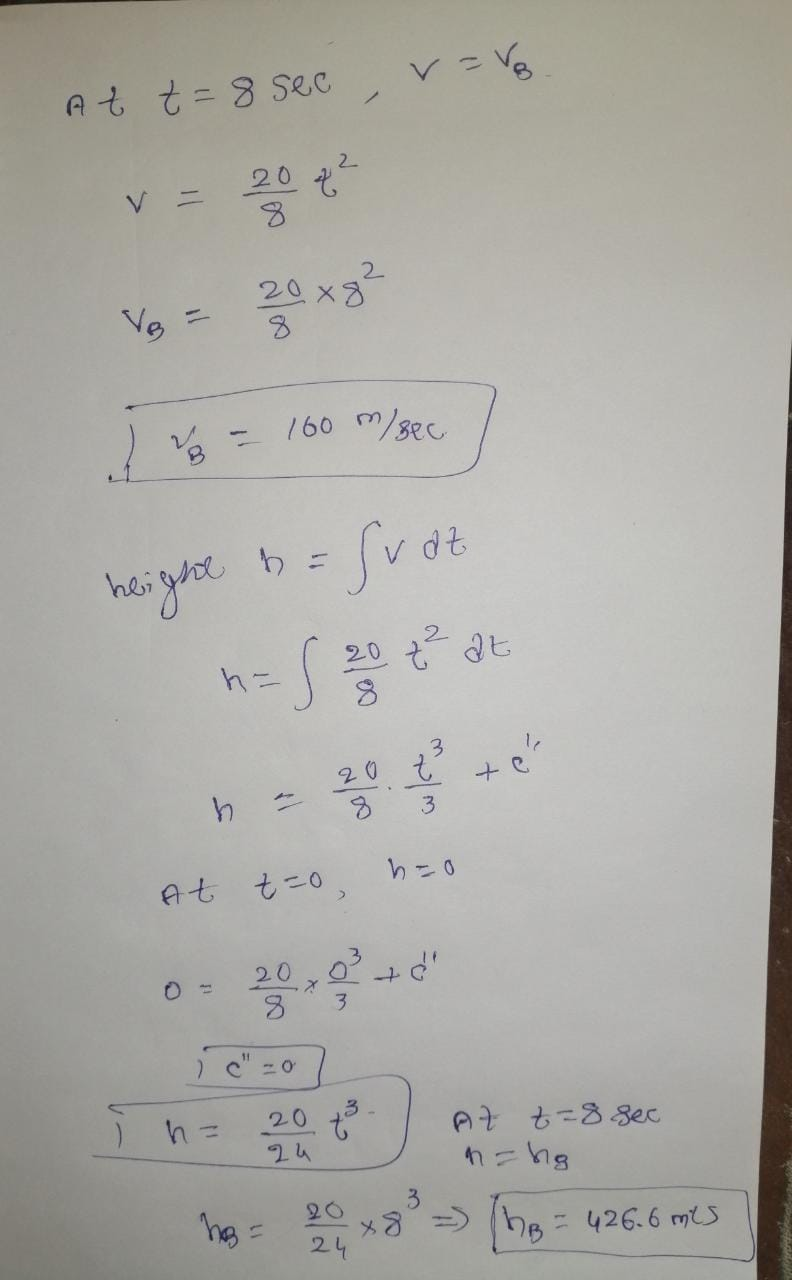"if you have any doubt at any point in this question, let me know in the comment section and I will help you for sure. Thank you and have a good day. Do upvote my answer if you like my explanation"

Earn Coins

Coins can be redeemed for fabulous gifts.

Similar Homework Help Questions
• The missile at A takes off from rest and rises vertically to B, where its fuel...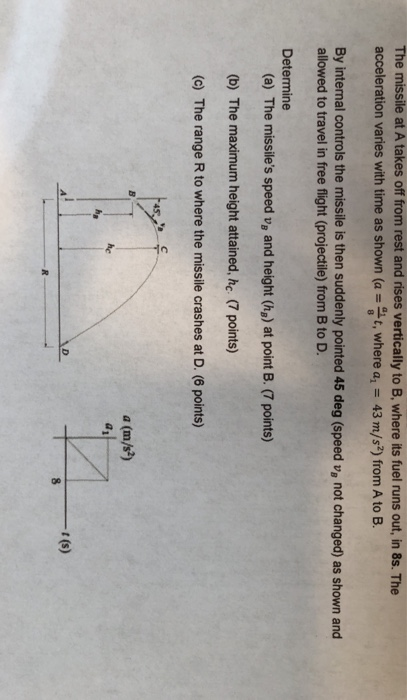The missile at A takes off from rest and rises vertically to B, where its fuel runs out, in 8s. The acceleration varies with time as shown (a = t, where a, = 43 m/s2) from A to B. By internal controls the missile is then suddenly pointed 45 deg (speed v, not changed) as shown and allowed to travel in free flight (projectile) from B to D. Determine (a) The missile's speed vg and height (hg) at point B....

• 12. During the first 30seconds of its flight, a test rocket's height in metres above its...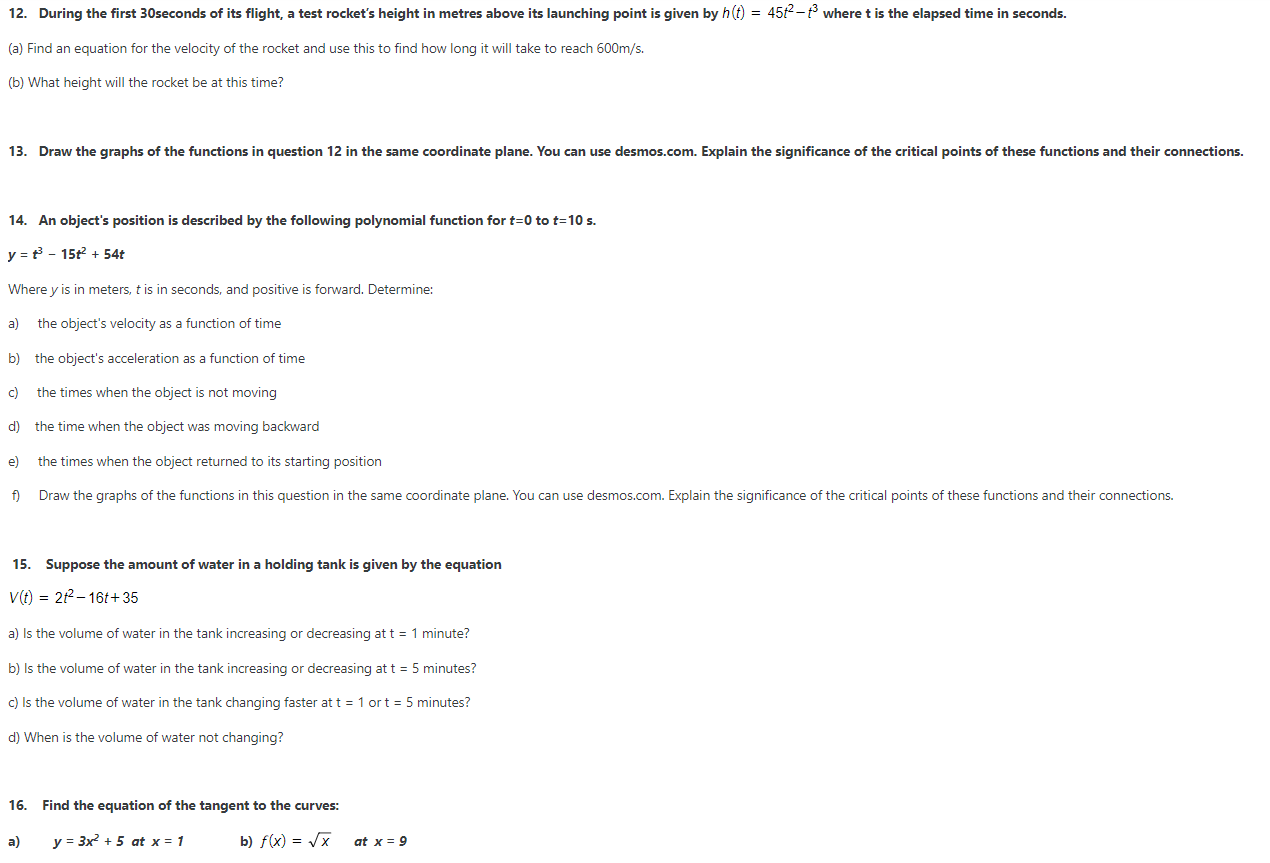12. During the first 30seconds of its flight, a test rocket's height in metres above its launching point is given by h(t) = 452 – where t is the elapsed time in seconds. (a) Find an equation for the velocity of the rocket and use this to find how long it will take to reach 600m/s. (b) What height will the rocket be at this time? 13. Draw the graphs of the functions in question 12 in the same coordinate...

• 75 To stop a car, first you require a certain reaction time to be 2The psition...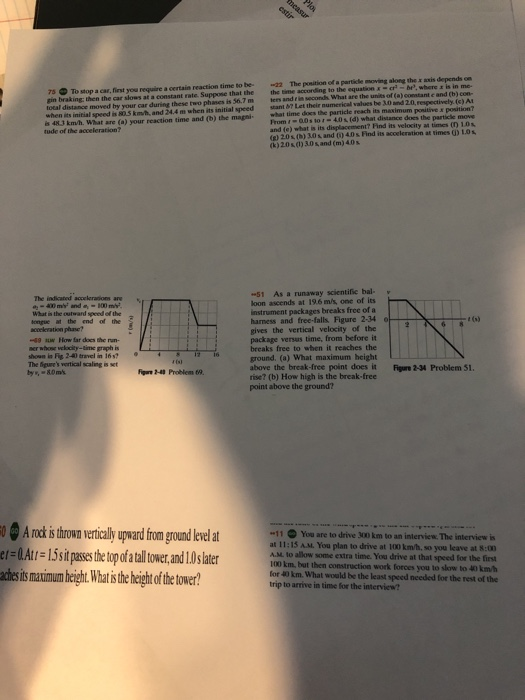75 To stop a car, first you require a certain reaction time to be 2The psition of a particle moving along the x sxis depends on gin braking: then the car slows at a constant rate. Suppose that the the time according to the equationxbP, where a is in me total distance moved by your car during these two phases is 56.7 m lers and rin secondh What are the units of (a) constant e and (b) con- when its...

• Thank you very much. College Physics (PHYC 2053 & 2 E3 Incorrect. You step onto a...Thank you very much. College Physics (PHYC 2053 & 2 E3 Incorrect. You step onto a hot beach time does it take for the impulse, which travels a distance of 1.65 m, to reach your brain? your bare feet. A nerve impulse, generated in your foot, travels through your nervous system at an average speed of 191 my's. How much ne Units m/s the tolerance is +/-4% LINK TO TEXT LINK TO TEXT Question Attempts: 1 of 3 used REP...

• please answer all prelab questions, 1-4. This is the prelab manual, just in case you need...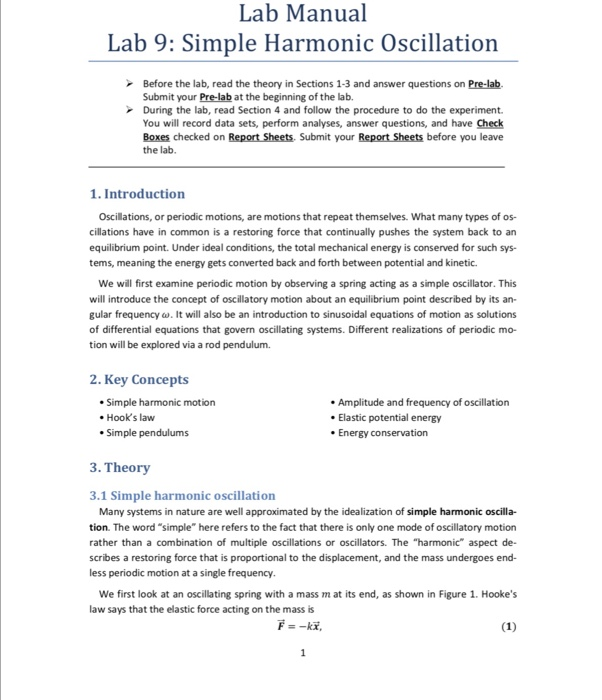please answer all prelab questions, 1-4. This is the prelab manual, just in case you need background information to answer the questions. The prelab questions are in the 3rd photo. this where we put in the answers, just to give you an idea. Lab Manual Lab 9: Simple Harmonic Oscillation Before the lab, read the theory in Sections 1-3 and answer questions on Pre-lab Submit your Pre-lab at the beginning of the lab. During the lab, read Section 4 and...

• How can we assess whether a project is a success or a failure? This case presents...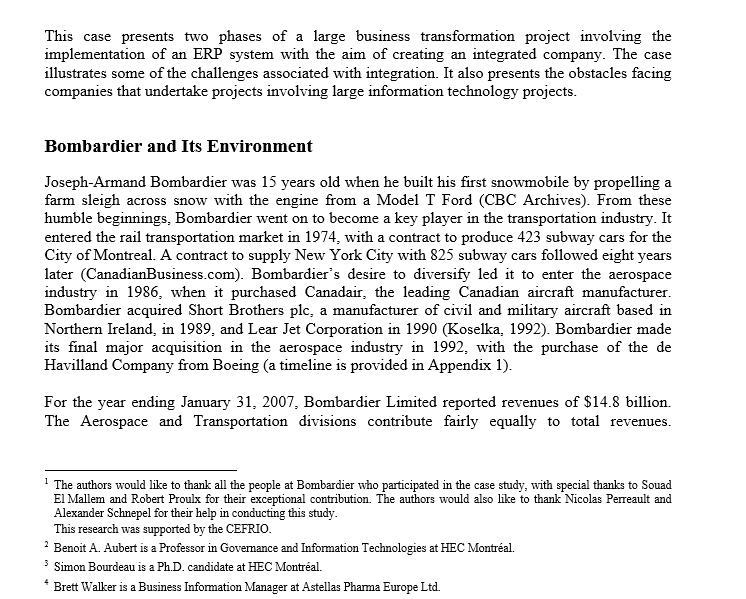How can we assess whether a project is a success or a failure? This case presents two phases of a large business transformation project involving the implementation of an ERP system with the aim of creating an integrated company. The case illustrates some of the challenges associated with integration. It also presents the obstacles facing companies that undertake projects involving large information technology projects. Bombardier and Its Environment Joseph-Armand Bombardier was 15 years old when he built his first snowmobile...